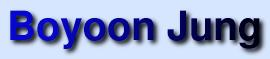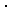Boyoon Jung's Library Manual

bj::ExtendedKalmanFilter Class Reference [Kalman Filter]

An Extended Kalman Filter class for non-linear systems. More...

#include <kalman.h>

List of all members.

Public Member Functions

ExtendedKalmanFilter (int stateDim, int inputDim, int *measurementDim, int size)
A constructor.
ExtendedKalmanFilter (int stateDim, int inputDim, int measurementDim)
A constructor.
ExtendedKalmanFilter (void)
A constructor.
~ExtendedKalmanFilter (void)
A destructor.
void initialize (gsl_vector *x, gsl_matrix *P, gsl_matrix *A=0, gsl_matrix *B=0, gsl_matrix *W=0, gsl_matrix *Q=0, gsl_matrix **H=0, gsl_matrix **V=0, gsl_matrix **R=0, int size=0)
Set parameters.
void intialize (gsl_vector *x, gsl_matrix *P, gsl_matrix *A, gsl_matrix *B, gsl_matrix *W, gsl_matrix *Q, gsl_matrix *H, gsl_matrix *V, gsl_matrix *R)
Set parameters.
void timeUpdate (gsl_vector *u, gsl_matrix *A=0, gsl_matrix *B=0, gsl_matrix *W=0, gsl_matrix *Q=0, double dt=0)
Evaluate the time update equation. [prediction step].
void timeUpdate (gsl_vector *u, double dt)
Evaluate the time update equation. [prediction step].
void measurementUpdate (gsl_vector *z, gsl_matrix *H=0, gsl_matrix *V=0, gsl_matrix *R=0, int index=0)
Evaluate the measurement update equation. [correction step].
void measurementUpdate (gsl_vector *z, int index)
Evaluate the measurement update equation. [correction step].
void update (gsl_vector *u, gsl_vector *z, gsl_matrix *A=0, gsl_matrix *B=0, gsl_matrix *W=0, gsl_matrix *Q=0, gsl_matrix *H=0, gsl_matrix *V=0, gsl_matrix *R=0, int index=0, double dt=0)
Evaluate the time & the measurement update equations.
void update (gsl_vector *u, gsl_vector *z, int index, double dt)
Evaluate the time & the measurement update equations.
void update (gsl_vector *u, gsl_vector *z, int index)
Evaluate the time & the measurement update equations.
void update (gsl_vector *u, gsl_vector *z, double dt)
Evaluate the time & the measurement update equations.
gsl_vector * state (void)
Retrieve the current state estimate.
gsl_matrix * covariance (void)
Retrieve the current error covariance.

Protected Member Functions

void gsl_matrix_inverse (gsl_matrix *org, gsl_matrix *inv, int index)
Compute the inverse matrix of a given square matrix.
virtual void f (gsl_vector *x, gsl_vector *u, gsl_vector *x_, double dt=0)=0
Define a non-linear transition matrix function.
virtual void h (gsl_vector *x_, gsl_vector *z, int index=0)=0
Define a non-linear measurement matrix function.

Protected Attributes

int msize
The number of measurements.
gsl_matrix * A
Jacobians for process model.
gsl_matrix * B
Jacobians for process model.
gsl_matrix * W
Jacobians for process noise model.
gsl_matrix * Q
Process noise model.
gsl_matrix ** H
Jacobians for measurement model.
gsl_matrix ** V
Jacobians for measurement noise model.
gsl_matrix ** R
Measurement noise model.
gsl_vector * x
State estimate.
gsl_matrix * P
Error covariance.
gsl_matrix ** K
Kalman gain.
gsl_permutation ** pi
int si
gsl_vector * t0_n1
gsl_matrix * t0_nn
gsl_matrix * t1_nn
gsl_vector ** t0_m1
gsl_matrix ** t0_mm
gsl_matrix ** t1_mm
gsl_matrix ** t2_mm
gsl_matrix ** t0_nm

Detailed Description

An Extended Kalman Filter class for non-linear systems.

• Dynamic equations:• Jacobian matrices:• Noise distribution:• Abstract functions:
• f(x, u, x_, dt) := process function x_ = f(x, u, dt)
• h(x_, z) := measurement function z = h(x_)

Constructor & Destructor Documentation

 bj::ExtendedKalmanFilter::ExtendedKalmanFilter ( int stateDim, int inputDim, int * measurementDim, int size )

A constructor.

This constructor is for when there are multiple measurements.

Parameters:
 stateDim The dimension of a state vector. inputDim The dimension of a control input vector. measurementDim The dimensions of measurement vectors. size The number of measurements.

 bj::ExtendedKalmanFilter::ExtendedKalmanFilter ( int stateDim, int inputDim, int measurementDim )

A constructor.

This constructor is for when there is a single measurement.

Parameters:
 stateDim The dimension of a state vector. inputDim The dimension of a control input vector. measurementDim The dimension of a measurement vector.

 bj::ExtendedKalmanFilter::ExtendedKalmanFilter ( void )
 A constructor.

 bj::ExtendedKalmanFilter::~ExtendedKalmanFilter ( void )
 A destructor.

Member Function Documentation

 gsl_matrix* bj::ExtendedKalmanFilter::covariance ( void ) [inline]
 Retrieve the current error covariance.

 virtual void bj::ExtendedKalmanFilter::f ( gsl_vector * x, gsl_vector * u, gsl_vector * x_, double dt = 0 ) [protected, pure virtual]
 Define a non-linear transition matrix function.

 void bj::ExtendedKalmanFilter::gsl_matrix_inverse ( gsl_matrix * org, gsl_matrix * inv, int index ) [inline, protected]
 Compute the inverse matrix of a given square matrix. The original matrix will be destroyed !!!

 virtual void bj::ExtendedKalmanFilter::h ( gsl_vector * x_, gsl_vector * z, int index = 0 ) [protected, pure virtual]
 Define a non-linear measurement matrix function.

 void bj::ExtendedKalmanFilter::initialize ( gsl_vector * x, gsl_matrix * P, gsl_matrix * A = 0, gsl_matrix * B = 0, gsl_matrix * W = 0, gsl_matrix * Q = 0, gsl_matrix ** H = 0, gsl_matrix ** V = 0, gsl_matrix ** R = 0, int size = 0 )

Set parameters.

This is for when there are multiple measurements.

Parameters:
 x Initial state estimate. P Initial error covariance. A Jacobian transition matrix. B Jacobian input matrix. W Jacobian system noise matrix. Q Covariance of process noise. H Jacobian measurement matrix. V Jacobian measurement noise matrix. R Covariance of measurement noise. size The number of measurements.

 void bj::ExtendedKalmanFilter::intialize ( gsl_vector * x, gsl_matrix * P, gsl_matrix * A, gsl_matrix * B, gsl_matrix * W, gsl_matrix * Q, gsl_matrix * H, gsl_matrix * V, gsl_matrix * R ) [inline]

Set parameters.

This is for when there is a single measurement.

Parameters:
 x Initial state estimate. P Initial error covariance. A Jacobian transition matrix. B Jacobian input matrix. W Jacobian system noise matrix. Q Covariance of process noise. H Jacobian measurement matrix. V Jacobian measurement noise matrix. R Covariance of measurement noise.

 void bj::ExtendedKalmanFilter::measurementUpdate ( gsl_vector * z, int index ) [inline]

Evaluate the measurement update equation. [correction step].

Parameters:
 z Measurement. index The index of a measurement when there are multiple measurements available.

 void bj::ExtendedKalmanFilter::measurementUpdate ( gsl_vector * z, gsl_matrix * H = 0, gsl_matrix * V = 0, gsl_matrix * R = 0, int index = 0 )

Evaluate the measurement update equation. [correction step].

Parameters:
 z Measurement. H Jacobian measurement matrix. V Jacobian measurement noise matrix. R Covariance of measurement noise. index The index of a measurement when there are multiple measurements available.

 gsl_vector* bj::ExtendedKalmanFilter::state ( void ) [inline]
 Retrieve the current state estimate.

 void bj::ExtendedKalmanFilter::timeUpdate ( gsl_vector * u, double dt ) [inline]

Evaluate the time update equation. [prediction step].

Parameters:
 u Control input. dt Time interval.

 void bj::ExtendedKalmanFilter::timeUpdate ( gsl_vector * u, gsl_matrix * A = 0, gsl_matrix * B = 0, gsl_matrix * W = 0, gsl_matrix * Q = 0, double dt = 0 )

Evaluate the time update equation. [prediction step].

Parameters:
 u Control input. A Jacobian transition matrix. B Jacobian input matrix. W Jacobian system noise matrix. Q Covariance of process noise. dt Time interval.

 void bj::ExtendedKalmanFilter::update ( gsl_vector * u, gsl_vector * z, double dt ) [inline]

Evaluate the time & the measurement update equations.

This function performs a full update cycle. It evaluate the time update equation first, and the measurement update is following.

Parameters:
 u Control input. z Measurement. dt Time interval.

 void bj::ExtendedKalmanFilter::update ( gsl_vector * u, gsl_vector * z, int index ) [inline]

Evaluate the time & the measurement update equations.

This function performs a full update cycle. It evaluate the time update equation first, and the measurement update is following.

Parameters:
 u Control input. z Measurement. index The index of a measurement.

 void bj::ExtendedKalmanFilter::update ( gsl_vector * u, gsl_vector * z, int index, double dt ) [inline]

Evaluate the time & the measurement update equations.

This function performs a full update cycle. It evaluate the time update equation first, and the measurement update is following.

Parameters:
 u Control input. z Measurement. index The index of a measurement. dt Time interval.

 void bj::ExtendedKalmanFilter::update ( gsl_vector * u, gsl_vector * z, gsl_matrix * A = 0, gsl_matrix * B = 0, gsl_matrix * W = 0, gsl_matrix * Q = 0, gsl_matrix * H = 0, gsl_matrix * V = 0, gsl_matrix * R = 0, int index = 0, double dt = 0 ) [inline]

Evaluate the time & the measurement update equations.

This function performs a full update cycle. It evaluate the time update equation first, and the measurement update is following.

Parameters:
 u Control input. z Measurement. A Jacobian transition matrix. B Jacobian input matrix. W Jacobian system noise matrix. Q Covariance of process noise. H Jacobian measurement matrix. V Jacobian measurement noise matrix. R Covariance of measurement noise. index The index of a measurement. dt Time interval.

Member Data Documentation

 gsl_matrix* bj::ExtendedKalmanFilter::A [protected]
 Jacobians for process model.

 gsl_matrix* bj::ExtendedKalmanFilter::B [protected]
 Jacobians for process model.

 gsl_matrix** bj::ExtendedKalmanFilter::H [protected]
 Jacobians for measurement model.

 gsl_matrix** bj::ExtendedKalmanFilter::K [protected]
 Kalman gain.

 int bj::ExtendedKalmanFilter::msize [protected]
 The number of measurements.

 gsl_matrix* bj::ExtendedKalmanFilter::P [protected]
 Error covariance.

 gsl_permutation** bj::ExtendedKalmanFilter::pi [protected]

 gsl_matrix* bj::ExtendedKalmanFilter::Q [protected]
 Process noise model.

 gsl_matrix** bj::ExtendedKalmanFilter::R [protected]
 Measurement noise model.

 int bj::ExtendedKalmanFilter::si [protected]

 gsl_vector** bj::ExtendedKalmanFilter::t0_m1 [protected]

 gsl_matrix** bj::ExtendedKalmanFilter::t0_mm [protected]

 gsl_vector* bj::ExtendedKalmanFilter::t0_n1 [protected]

 gsl_matrix** bj::ExtendedKalmanFilter::t0_nm [protected]

 gsl_matrix* bj::ExtendedKalmanFilter::t0_nn [protected]

 gsl_matrix ** bj::ExtendedKalmanFilter::t1_mm [protected]

 gsl_matrix * bj::ExtendedKalmanFilter::t1_nn [protected]

 gsl_matrix ** bj::ExtendedKalmanFilter::t2_mm [protected]

 gsl_matrix** bj::ExtendedKalmanFilter::V [protected]
 Jacobians for measurement noise model.

 gsl_matrix* bj::ExtendedKalmanFilter::W [protected]
 Jacobians for process noise model.

 gsl_vector* bj::ExtendedKalmanFilter::x [protected]
 State estimate.

The documentation for this class was generated from the following file:
ResearchProjectsPublications

ToolsBjLib ManualPlayer/StagePyPlayerMicroStrain IMUGPS/DGPSParticle Filter

PersonalWelcomeCurriculum VitaeResumeContactUSCUSC CS DeptCRESRobotics LabRESL